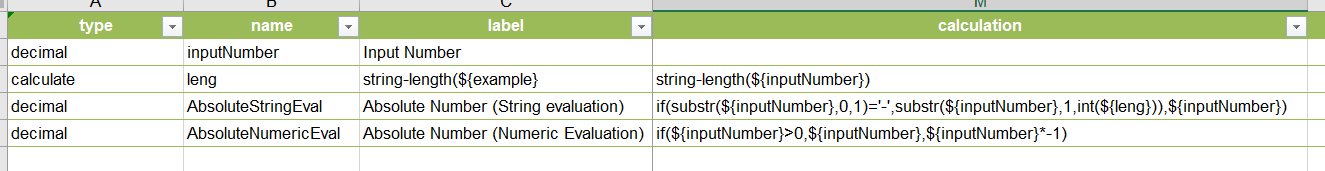# Does Survey123 support the absolute value function?

968
8
05-31-2017 07:48 AM
Highlighted

Hello,

Is it possible use the absolute value function in survey123? I need to formulate a logic statement based on the weight difference of two things. Example: "If the difference is > 0.3, the test fails." I have not seen any examples online showing absolute value. Any solutions or work arounds you know of would be greatly appreciated.

Thank you!

Tags (3)
1 Solution

Accepted Solutions
Highlighted
byEsri Frequent ContributorIn the absence of the abs() function, will the above work for you?

You could keep the Calcu question as a 'calculate' and then use that variable in other expressions.

8 Replies
Highlighted
byEsri Frequent ContributorIn the absence of the abs() function, will the above work for you?

You could keep the Calcu question as a 'calculate' and then use that variable in other expressions.

Highlighted

This worked!!! Thank you so much!! Could you explain the language of the calculation in the 3rd row or point me toward a resource that would explain it? I'd like to understand it a bit further. Thank you!!!

Highlighted
byEsri Esteemed Contributor

Hi Kara,

The statement says:

1) Treat the answer as text and look at the first character of the answer.  See if it is a minus sign ( - )

2) If yes, grab everything in the answer except for the minus sign (from character in position 1 - *the second character* - to the end of the answer) and make that the value

3) In not, just give value of the question being looked at

the equivalent using numeric evaluation would be

if(\${question} < 0, \${question} * -1, \${question})

Highlighted

Thank you so much!

Highlighted
byEsri Frequent Contributor

Hi Kara.

I used operators and functions as described in this help topic: Formulas—Survey123 for ArcGIS | ArcGIS

Essentially:

• substr() is used to extract portions of a string. I use  it for  example to extract the first character, which in our  case  could be either  a minus sign (which we  will want to remove later) or a digit. This function takes three inputs:
• The string from which you want to extract a substring
• The character position from which you want to start substracing
• The number of characters to remove
• string-length() is  used to calculate the total length of a string.
• if(). the if statement allows  you evaluate an expression. If the expression evaluates to true, you can output one value, and if it evaluates to false, you output another value.

There is no single way to solve this, as shown by James above. There may be other ways to solve it too.

Highlighted

Thank you!

Highlighted

Just for future reference, if anyone comes across this...

Under the calculations section they talk about the substr function.

Highlighted
Occasional Contributor

If you want to calculate it in a single Calculation field, you can also do the following:

sqrt(pow(\${number1} - \${number2},2))

I squared the number and then took the square root. The result will always be positive.